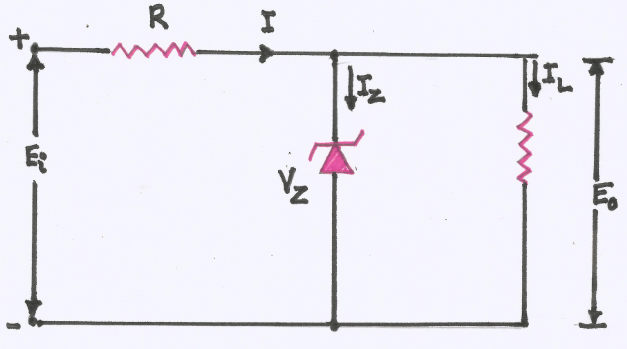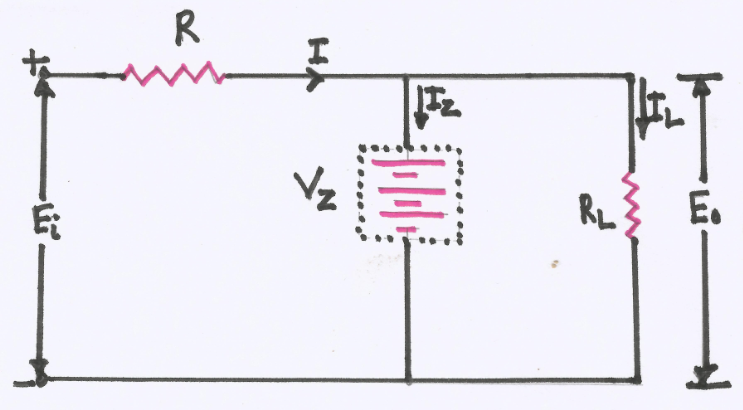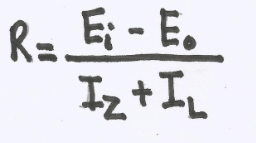# Explain with a circuit diagram, the use of a Zener diode as a reference diode

## Zener diode as a reference diode

A Zener diode can be used as a voltage regulator to provide a constant voltage from a source whose voltage may vary over sufficient range. The circuit arrangement is shown in the figure below:The Zener diode of Zener voltage VZ is reverse connected across the load RL across which constant output is desired. The series resistance R absorbs the output voltage fluctuations so as to maintain constant voltage across the load. It may be noted that the Zener will maintain a constant voltage VZ (=E0) across the load so long as the input voltage does not fall below VZ.

When the circuit is properly designed, the load voltage E0 remains essentially constant (equal to VZ) even though the input voltage Ei and load resistance RL may vary over a wide range.

### Case 1 : Input Voltage Increases

Suppose the input voltage increases. Since the Zener is in the breakdown region, the Zener diode is equivalent to a battery VZ as shown in the figure below:It is clear that output voltage remains constant at VZ(~ E0). The excess voltage is dropped across the series resistance R. This will cause an increase in the value of total current I. The Zener will conduct excess current while the load current remains constant. Hence, output voltage E0 remains constant irrespective of the changes in the input voltage Ei.

### Case 2 : Load Resistance Decreases

Now suppose that input voltage is constant but the load resistance RL decreases. This will cause an increase in load current. The extra current cannot come from the source because drop in R (and hence source current I) will not change as the Zener within its regulating range. The additional load current will come from a decrease in Zener current IZ. Consequently, the output voltage stays at constant value.

Voltage drop across R = Ei – Eo

Current through R, I = IZ + IL

Applying ohm’s law we have,